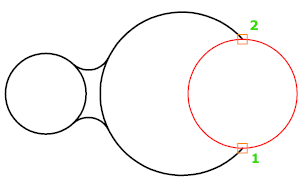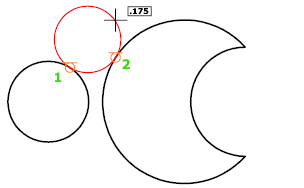# CIRCLE (Command)

Creates a circle.

Find

The following prompts are displayed.

### Center Point

Creates a circle based on a center point and a radius or diameter value.

Enter a value, or specify a point.

For example: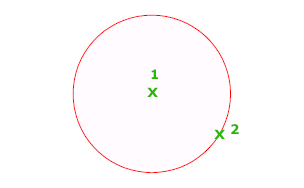Diameter

Enter a value, or specify a second point.

For example: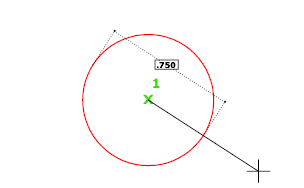### 3P (Three Points)

Creates a circle based on three points on the circumference.

For example: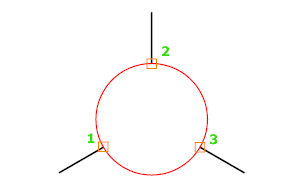### Tan, Tan, Tan

Creates a circle tangent to three objects.

For example: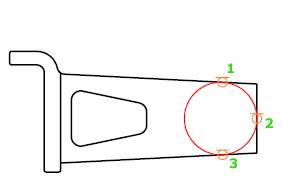### 2P (Two Points)

Creates a circle based on two endpoints of the diameter.

For example: